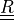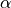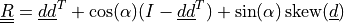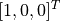# sfepy.linalg.geometry module¶

sfepy.linalg.geometry.barycentric_coors(coors, s_coors)[source]

Get barycentric (area in 2D, volume in 3D) coordinates of points with coordinates coors w.r.t. the simplex given by s_coors.

Returns:
bcarray

The barycentric coordinates. Then reference element coordinates xi = dot(bc.T, ref_coors).

sfepy.linalg.geometry.flag_points_in_polygon2d(polygon, coors)[source]

Test if points are in a 2D polygon.

Parameters:
polygonarray, (:, 2)

The polygon coordinates.

coors: array, (:, 2)

The coordinates of points.

Returns:
flagbool array

The flag that is True for points that are in the polygon.

Notes

This is a semi-vectorized version of .

 PNPOLY - Point Inclusion in Polygon Test, W. Randolph Franklin (WRF)

Return indices of coordinates inside or outside a ball given by centre and radius.

Notes

All float comparisons are done using <= or >= operators, i.e. the points on the boundaries are taken into account.

Return indices of coordinates inside a tube given by centre, axis vector, inner and outer radii and length.

Parameters:

If False, select points outside the radii, but within the tube length.

Notes

All float comparisons are done using <= or >= operators, i.e. the points on the boundaries are taken into account.

sfepy.linalg.geometry.get_face_areas(faces, coors)[source]

Get areas of planar convex faces in 2D and 3D.

Parameters:
facesarray, shape (n, m)

The indices of n faces with m vertices into coors.

coorsarray

The coordinates of face vertices.

Returns:
areasarray

The areas of the faces.

sfepy.linalg.geometry.get_perpendiculars(vec)[source]

For a given vector, get a unit vector perpendicular to it in 2D, or get two mutually perpendicular unit vectors perpendicular to it in 3D.

sfepy.linalg.geometry.get_simplex_circumcentres(coors, force_inside_eps=None)[source]

Compute the circumcentres of n_s simplices in 1D, 2D and 3D.

Parameters:
coorsarray

The coordinates of the simplices with n_v vertices given in an array of shape (n_s, n_v, dim), where dim is the space dimension and 2 <= n_v <= (dim + 1).

force_inside_epsfloat, optional

If not None, move the circumcentres that are outside of their simplices or closer to their boundary then force_inside_eps so that they are inside the simplices at the distance given by force_inside_eps. It is ignored for edges.

Returns:
centresarray

The circumcentre coordinates as an array of shape (n_s, dim).

sfepy.linalg.geometry.get_simplex_volumes(cells, coors)[source]

Get volumes of simplices in nD.

Parameters:
cellsarray, shape (n, d)

The indices of n simplices with d vertices into coors.

coorsarray

The coordinates of simplex vertices.

Returns:
volumesarray

The volumes of the simplices.

sfepy.linalg.geometry.inverse_element_mapping(coors, e_coors, eval_base, ref_coors, suppress_errors=False)[source]

Given spatial element coordinates, find the inverse mapping for points with coordinats X = X(xi), i.e. xi = xi(X).

Returns:
xiarray

The reference element coordinates.

sfepy.linalg.geometry.make_axis_rotation_matrix(direction, angle)[source]

Create a rotation matrixcorresponding to the rotation around a general axisby a specified angle.Parameters:
directionarray

The rotation axis direction vector.

anglefloat

The rotation angle.

Returns:
mtxarray

The rotation matrix.

Notes

The matrix follows the right hand rule: if the right hand thumb points along the axis vectorthe fingers show the positive angle rotation direction.

Examples

Make transformation matrix for rotation of coordinate system by 90 degrees around ‘z’ axis.

>>> mtx = make_axis_rotation_matrix([0., 0., 1.], nm.pi/2)
>>> mtx
array([[ 0.,  1.,  0.],
[-1.,  0.,  0.],
[ 0.,  0.,  1.]])


Coordinates of vectorw.r.t. the original system in the rotated system. (Or rotation of the vector by -90 degrees in the original system.)

>>> nm.dot(mtx, [1., 0., 0.])
>>> array([ 0., -1.,  0.])


Coordinates of vectorw.r.t. the rotated system in the original system. (Or rotation of the vector by +90 degrees in the original system.)

>>> nm.dot(mtx.T, [1., 0., 0.])
>>> array([ 0.,  1.,  0.])

sfepy.linalg.geometry.points_in_simplex(coors, s_coors, eps=1e-08)[source]

Test if points with coordinates coors are in the simplex given by s_coors.

sfepy.linalg.geometry.rotation_matrix2d(angle)[source]

Construct a 2D (plane) rotation matrix corresponding to angle.

sfepy.linalg.geometry.transform_bar_to_space_coors(bar_coors, coors)[source]

Transform barycentric coordinates bar_coors within simplices with vertex coordinates coors to space coordinates.